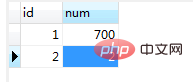# 介绍thinkphp lock锁的使用和例子```class Index extends Controller
{
public function index()
{

\$model=Db::name('test');
\$model->startTrans();
try{
\$list=\$model->lock(true)->find();
\$model->where(['id'=>1])->data(['num'=>900])->update();//id为1的更新
sleep(10);//等待10秒
\$model->commit();
print_r(\$list);
}catch (\Exception \$exception){
\$model->rollback();
throw \$exception;

}

}

public function index2(){

\$model=Db::name('test');
\$model->startTrans();
try{
\$list=\$model->lock(true)->where(['id'=>1])->find();//id为1在更新时，select id=1 会等待。把ID改为2时，不等待
\$model->commit();
print_r(\$list);
}catch (\Exception \$exception){
\$model->rollback();
throw \$exception;

}

}
}```